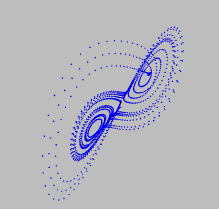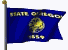# Lorentz Attractor

by Kirby Urner
First posted: Nov 15, 2001```"""
Adapted from Flash 5 movie by jtarbell, March 2001,
levitated.net, based on the work of Ed N. Lorenz

by K. Urner, Oregon Curriculum Network, November, 2001
"""

import math, coords, povray

class Lorentz:

def __init__(self,iterations=2000,x0=0,y0=-2,z0=-1):
self.num = iterations
# initialize seed
# strange thing is, these numbers can be just about anything
# yet they will always converge on an identical solution set
self.x0 = x0
self.y0 = y0
self.z0 = z0
self.depths = {}
self.outfile = \
povray.Povray("lorentz2.pov",cf=25.0,cx=0,cy=0,bgcolor="Gray")
self.outfile.sphcolor = "Blue"

def run(self):
# lorentz constants - don't change these
h = 0.01
a = 10.0
b = 28.0
c = 8.0/3.0

x0 = self.x0
y0 = self.y0
z0 = self.z0

n = 0

while (n < self.num):
n += 1
# lorentz linear function set
x1 = x0 + h *  a*(y0-x0)
y1 = y0 + h * (x0*(b-z0) - y0)
z1 = z0 + h * (x0*y0 - c*z0)
# solution becomes next seed
x0 = x1
y0 = y1
z0 = z1
# write sphere to Povray file
v = coords.Vector([x0,y0,z0])
v = v*0.1 # re scale
self.outfile.point(v)

self.outfile.close()

```

Example usage pattern:

```>>> import lorentz
>>> ol = lorentz.Lorentz()
>>> ol.run()
```

Then open Povray and render lorentz2.pov (which file name you can of course change by modifying the code and/or making a user-supplied value). The result I got from running the above (slightly cropped) is displayed up top.

A fun project would be to modify the above to have it run in real time, adding points as the viewer watches, perhaps in tandem with VPython, which provides easy access to a dynamic rendering window. Or you could go directly to PyOpenGL (see links below).

 For further reading: Lorentz Attractor @ levitated.net (Flash movie) Numeracy + Computer Literacy essay (explains povray, coords modules) VPython PyOpenGL Fractals page (more simple source) More Plotting with PIL Above source code in .py (text) format Return to CP4EOregon Curriculum Network